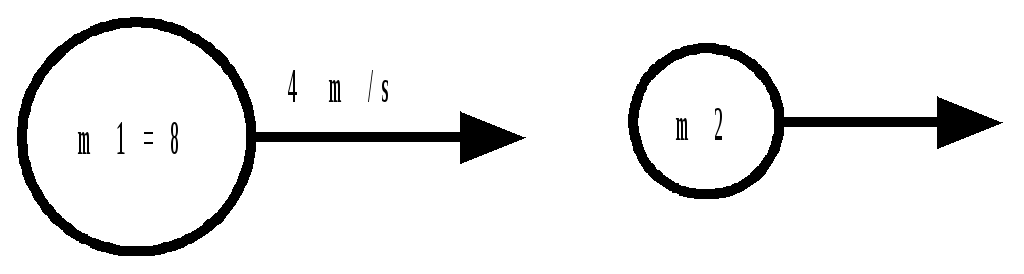# Momentum and Collisions

 Date 28.05.2018 Size 41.12 Kb. #52220
Momentum and Collisions

1. A 20.0 kg ball with velocity of 20.0 m/s East strikes a 2.00 kg ball that is initially at rest. After collision, the velocity of the 20.0 kg ball has decreased to 15 m/s. Find the final velocity of the 2.00 kg ball.

2. An 8.00 kg ball traveling at 4.00 m/s strikes another ball M2. After collision, M1 is traveling in the same direction but at only 3.00 m/s, and M2 is traveling at 4.00 m/s. Find the mass of M2.

1. A 4.00 kg ball with a velocity of 4.00 m/s East, overtakes a 2.00 kg ball traveling at 2.00 m/s East. If the final velocity of the 4.00 kg ball is 3.00 m/s, find the final velocity of the 2.00 kg ball.

1. A 10.00 kg ball traveling at +6.00 m/s overtakes a 5.00 kg ball traveling at +4.00 m/s. If the 10.0 kg ball slows down to +3.00 m/s after the collision, find the final velocity of the 5.00 kg ball.

1. A 20.0 kg ball with V = 5.00 m/s East strikes a 2.00 kg ball with velocity 10.0 m/s West. If the final speed of the 20.0 kg ball is 3.00 m/s East, what is the final velocity of the other ball?

1. A 6.00 kg ball traveling East at 2.00 m/s strikes ball B of mass 2.00 kg at rest. If they stick together after collision, what is their final velocity?7. When the 80.0 kg football player, who is standing on a frictionless surface, catches a 4 kg football moving at 30 m/s, what will be his final velocity?

1. A 3.00 kg mass moving at 2.00 m/s North strikes a 1.00 kg mass at rest. After the collision, the 3.00 kg mass moves at 1.00 m/s at 60 West of North. Find the velocity and direction of the 1.00 kg mass. Assume the collision is perfectly elastic.

1. Ball A, of mass 4.00 kg and heading North at 2.00 m/s, strikes a 2.00 kg Ball B which is at rest. After collision, Ball A is traveling at 1.50 m/s at 55 North of East. Find the speed and direction of Ball B.

1. Two masses, A and B, which are both moving, collide with one another:

Before collision: A: 3.00 kg at 2.00 m/s North West

B: 4.00 kg at 3.00 m/s North East

After collision: B: 4.00 kg at 2.00 m/s North
Find the speed and direction of mass A.

1. Two masses, A and B, which are both moving, collide with one another:

Before collision: A: 2.00 kg at 2.50 m/s North

## B: 3.00 kg at 2.00 m/s 20 West of North

After collision: A: 2.00 kg at 2.00 m/s North West
Find the speed and direction of Ball B.

1. Vr1 = 50 m/s

2. m2 = 2 kg

3. V21 = 4 m/s

4. V21 = 10 m/s

5. V21 = 10 m/s

6. V1 = 1.5 m/s

7. V1 = 1.43

8. V1 = 5.2 m/s at 30 E of N

9. V1 = 2.65 m/s at N 35 W

10. VA1 = 2.13 m/s E 48 N

11. V = 2.6 m/s at 5 E of N

See http://cabennet.mvsd.ca/PHYS40S/Momentum/momentum and collisions solns.pdf for solutions